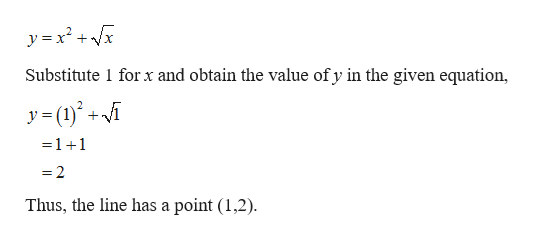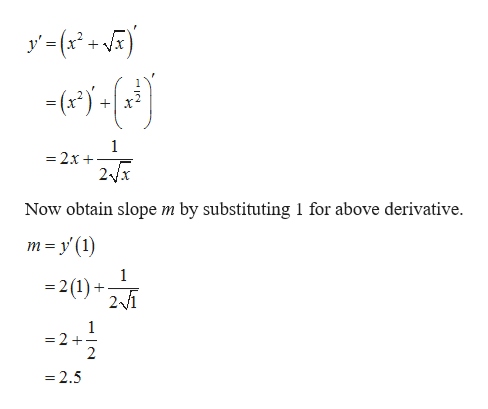Find the equation of the tangent line to the curve y = x^2 + sqrt(x) at a = 1.

Question

Find the equation of the tangent line to the curve y = x^2 + sqrt(x) at a = 1.

Step 1

Given function is,help_outlineImage Transcriptionclosey x2x Substitute 1 forx and obtain the value of y in the given equation, y(i - 1 +1 2 Thus, the line has a point (1,2) fullscreen
Step 2

The slope of the tangent at a...help_outlineImage Transcriptionclosey'(x + 1 =2x 2Vx Now obtain slope m by substituting 1 for above derivative m y (1) 1 - 2(1) + 1 = 2 + 2 -2.5 fullscreen

Want to see the full answer?

See Solution

Want to see this answer and more?

Our solutions are written by experts, many with advanced degrees, and available 24/7

See Solution
Tagged in

Other